Fluid Mechanics MCQ Level -1

# Fluid Mechanics MCQ Level -1

Test Description

## 40 Questions MCQ Test | Fluid Mechanics MCQ Level -1

Fluid Mechanics MCQ Level -1 for Class 11 2022 is part of Class 11 preparation. The Fluid Mechanics MCQ Level -1 questions and answers have been prepared according to the Class 11 exam syllabus.The Fluid Mechanics MCQ Level -1 MCQs are made for Class 11 2022 Exam. Find important definitions, questions, notes, meanings, examples, exercises, MCQs and online tests for Fluid Mechanics MCQ Level -1 below.
Solutions of Fluid Mechanics MCQ Level -1 questions in English are available as part of our course for Class 11 & Fluid Mechanics MCQ Level -1 solutions in Hindi for Class 11 course. Download more important topics, notes, lectures and mock test series for Class 11 Exam by signing up for free. Attempt Fluid Mechanics MCQ Level -1 | 40 questions in 40 minutes | Mock test for Class 11 preparation | Free important questions MCQ to study for Class 11 Exam | Download free PDF with solutions
 1 Crore+ students have signed up on EduRev. Have you?
Fluid Mechanics MCQ Level -1 - Question 1

### A large wooden piece in the form of a cylinder floats onwater with two-thirds of its length immersed. When aman stands on its upper surface, a further one-sixth ofits length is increased. The ratio between the massesof the man and the wooden piece is

Fluid Mechanics MCQ Level -1 - Question 2

### A wooden block in the form of a uniform cylinder floatswith one-third length above the water surface. A smallchip of this block is held at rest at the bottom of acontainer containing water to a height of 1 m and isthen released. The time in which it will rise to the surfaceof water is (g = 10 m/s2)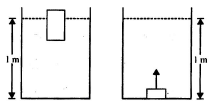Fluid Mechanics MCQ Level -1 - Question 3

### A goldsmith desires to test the purity of a gold ornamentsuspected to the mixed with copper. The ornamentweighs 0.25 kg in air and is observed to displace 0.015litre of water when immersed in it. Densities of goldand copper with respect to water are, respectively, 19.3and 8.9. The approximate percentage of copper in theornament is

Fluid Mechanics MCQ Level -1 - Question 4

An ice cube is floating in water above which a layer ofa lighter oil is poured. As the ice melts completely, thelevel of water surface (or interface) and upper level ofoil will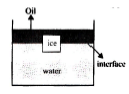Fluid Mechanics MCQ Level -1 - Question 5

In the figure shown the velocity and pressure of theliquid at the cross section (2) are given by (If P0 is theatmospheric pressure).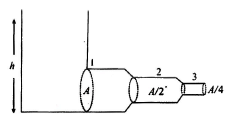Fluid Mechanics MCQ Level -1 - Question 6

A tube filled with water and closed at both ends uniformlyrotates in a horizontal plane about the OO’ axis. Themanometers A and B fixed in the tube at distancesr1 and r2 from rotational axis indicate pressures p1 andp2 respectively. Determine the angular velocity ω ofrotation of the tube  .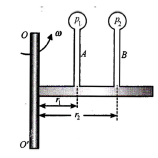Fluid Mechanics MCQ Level -1 - Question 7

A horizontal tube has different cross sections at pointsA and B. The areas of cross section are a1 and a2,respectively, and pressures at these points arep1 = pgh1 and p2 = pgh2, where p is the density of liquidflowing in the tube and h1, and h2 are heights of liquidcolumns in vertical tubes connected at A and B. Ifh1- h2 = h2 then the flow rate of the liquid in the horizontaltube is

Fluid Mechanics MCQ Level -1 - Question 8

Figure shows a crude type of atomizer. When bulb A iscompressed, air flows swiftly through tube BC causinga reduced pressure in the particles of the vertical tube.Liquid rises in the tube, enters BC and is sprayed out.If the pressure in the bulb is Pa + P, where P is thegauge pressure and Pa is the atmospheric pressure, vis the speed of air in BaC, find how large would v needto be to cause the liquid to rise to BC. Density of air =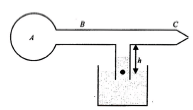Fluid Mechanics MCQ Level -1 - Question 9

A cubic block of side a is connected with two similarvertical springs as shown. Initially, bottom surface ofthe block of density a touches the surface of the fluidof density 2σ while floating. A weight is placed on theblock so that it is immersed half in the fluid, find theweight.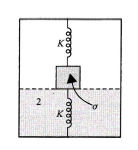Fluid Mechanics MCQ Level -1 - Question 10

50Water from a tap emerges vertically downwards withan initial velocity V0. Assuming pressure is constantthroughout the stream of water and the flow is steady,find the distance from the tap at which cross-sectionalarea of stream is half of the cross-sectional area ofstream at the tap:

Fluid Mechanics MCQ Level -1 - Question 11

A large open tank has two holes in the wall. One is asquare hole of side L at a depth y from the top and theother is a circular hole of radius R at a depth 4y fromthe top. When the tank is completely filled with water,the quantities of water flowing out per second from boththe holes are the same. Then, R is equal to

Fluid Mechanics MCQ Level -1 - Question 12

A U-shaped tube contains a liquid of density r and it is rotated about the left dotted line as shown in the figure. Find the difference in the levels of the liquid column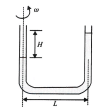Fluid Mechanics MCQ Level -1 - Question 13

In the arrangement as shown, A and B are two cylinderseach of length L and density d1 and d2 (d1 < d2),respectively. They are joined together and submergedin the liquid of density d with length L/2 of A outsidethe liquid. If the difference in densities of two cylindersis equal to density of the liquid, find d1.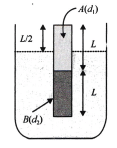Fluid Mechanics MCQ Level -1 - Question 14

The ratio of diameters of two wires of same material isn:l. The length of each wire is 4 m. On applying thesame load, the increases in the length of the thin wire will be (n > 1)

Fluid Mechanics MCQ Level -1 - Question 15

The dimensions of four wires of the same material aregiven below, In which wire the increase in the lengthwill be maximum?

Fluid Mechanics MCQ Level -1 - Question 16

Two wires of the same material and length but diametersin the ratio 1:2 are stretched by the same force. Thepotential energy per unit volume for the two wires whenstretched will be in the ratioa.

Fluid Mechanics MCQ Level -1 - Question 17

Two wires of the same length and same material butradii in the ratio of 1:2 are stretched by unequal forcestoproduce equal elongation. The ratio of the two forces is

Fluid Mechanics MCQ Level -1 - Question 18

58When a weight of 5 kg is suspended from a copperwire of length 30 m and diameter 0.5 mm, the length ofthe wire increases by 2.4 cm. If the diameter is doubled,the extension produced is

Fluid Mechanics MCQ Level -1 - Question 19

An elastic material of Young’s modulus Y is subjectedto a-stress S. The elastic energy stored per unit volumeof the material is

Fluid Mechanics MCQ Level -1 - Question 20

60What amount of work is done in increasing the lengthof a wire through unity?

Fluid Mechanics MCQ Level -1 - Question 21

Two wires of the same material have lengths in theratio 1:2 and their radii are in the ratio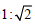. If theyare stretched by applying equal forces, the increase intheir lengths will be in the ratio

Fluid Mechanics MCQ Level -1 - Question 22

A piece of copper wire has twice the radius of a pieceof steel wire. Young’s modulus for steel is twice that ofthe copper. One end of the copper wire is joined to oneend of the steel wire so that both can be subjected tothe same longitudinal force. By what fraction of its lengthwill the steel have stretched when the length of thecopper has increased by 1%?

Fluid Mechanics MCQ Level -1 - Question 23

The velocity of small ball of mass M and density d1 =when dropped a container filled with glycerine becomesconstant after some time. If the density glycerine is d2,the viscous force acting on ball is

Fluid Mechanics MCQ Level -1 - Question 24

A large number of droplets, each of radius a. coalesce to form a bigger drop of radius b. Assume that the energy released in the process is converted into the kinetic energy of the drop. The velocity of the drop is (σ = surface tension, ρ = density)

Fluid Mechanics MCQ Level -1 - Question 25

A thick rope of density p and length L is hung from arigid support. The increase in length of the rope due toits own weight is (K is the Young’s modulus)

Fluid Mechanics MCQ Level -1 - Question 26

66When the load on a wire is slowly increased from 3 to 5 kg wt, the elongation increases from 0.61 to 1.02 mm. The work done during the extension of wire is

Fluid Mechanics MCQ Level -1 - Question 27

A wire of cross section A is stretched horizontallybetween two clamps located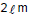apart. A weight Wkg is suspended from the mid-point of the wire. If themid-point sags vertically through a distance x < 1 the strain produced

Fluid Mechanics MCQ Level -1 - Question 28

If in the above question, the Young’s modulus of thematerial is Y, the value of extension x is

Fluid Mechanics MCQ Level -1 - Question 29

One end of uniform wire of length L and of weight W isattached rigidly to a point in the roof and a weight W,is suspended from its lower end. If s is the area ofcross section of the wire, the stress in the wire at aheight (3L/4) from its lower end is

Fluid Mechanics MCQ Level -1 - Question 30

Young’s modulis of brass and steel are 10 x 10 N/mand 2 x 10" N/m2, respectively. A brass wire and asteel wire of the same length are extended by 1 mmunder the same force. The radii of the brass and steelwires are RB and Rs, respectively. Then

Fluid Mechanics MCQ Level -1 - Question 31

When the tension in a metal wire is T1, its length is /1When the tension is T2, its length is /2. The naturallength of wire is

Fluid Mechanics MCQ Level -1 - Question 32

Two blocks of masses 1 kg and 2 kg are connected bya metal wire going over a smooth pulley as shown inthe figure. The breaking stress of the metal is (40/3 p)x 106 N/m2. If g = 10 m/s2, then what should be theminimum radius of the wire used if it is not to break?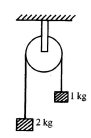Fluid Mechanics MCQ Level -1 - Question 33

If the work done by stretching a wire by I mm is 2 J.the work necessary for stretching another wire of thesame’ material but with the radius of cross sectionand half the length by 1 mm is

Fluid Mechanics MCQ Level -1 - Question 34

Two wires of the same material and same mass arestretched by the same force. Their lengths are in theratio 2:3.Their elongations are in the ratio

Fluid Mechanics MCQ Level -1 - Question 35

Two bars A and B of circular cross section, same volumeand made of the same material, are subjected totension. If the diameter of A is half that of B and if theforce applied to both the rod is the same and it is inthe elastic limit, the ratio of extension of A to that of Bwill be

Fluid Mechanics MCQ Level -1 - Question 36

Two glass plates are separated by water. If surfacetension of water is 75 dyn/cm and the area of eachplate wetted by water is 8 cm2 and the distancebetween the plates is 0.12 mm. then the force appliedto separate the two plates is

Fluid Mechanics MCQ Level -1 - Question 37

A soap film of surface tension 3 x 10-2 formed in arectangular frame can support a straw as shown in thefigure. If g = 10 m/s2, the mass of the straw is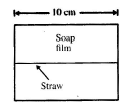Fluid Mechanics MCQ Level -1 - Question 38

A number of droplets, each of radius r, combine to form a drop of radius R. If T is the surface tension, the rise in temperature will be

Fluid Mechanics MCQ Level -1 - Question 39

A drop of liquid of density ρ is floating half-immersedina liquid of density d. If ρ is the surface tension thediameter of the drop of the liquid is

Fluid Mechanics MCQ Level -1 - Question 40

A drop of water of volume V is pressed between the twoglass palced so as to spread to an area A. If T is thesurface tension, the normal force required to separatethe glass plates is

 Use Code STAYHOME200 and get INR 200 additional OFF Use Coupon Code
Information about Fluid Mechanics MCQ Level -1 Page
In this test you can find the Exam questions for Fluid Mechanics MCQ Level -1 solved & explained in the simplest way possible. Besides giving Questions and answers for Fluid Mechanics MCQ Level -1 , EduRev gives you an ample number of Online tests for practice